# OpenFOAM® v1806: New and improved numerics

29/06/2018

## New scheme stabilisation

A new function object, stabilityBlendingFactor, enables automatic calculation of a blending factor between two discretisation schemes. This can be used to stabilise (steady) calculations on lower quality meshes or vice versa allow use of higher order discretisation where allowable.

The function object calculates the stabilityBlendingFactor field to be used by the localBlended convection scheme. The output is a surface-based weight field between 0-1, indicating the amount of the first scheme in the localBlended definition. In general the first scheme is the more stable, lower order scheme and the second the less stable but higher order scheme.

The weight,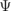, of a blended scheme is given by a function of the blending factor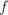: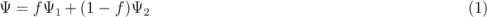where <scheme1> and <scheme2> are the first and second schemes in the localBlended entry (in the divSchemes part of the fvSchemes dictionary), e.g.

div(phi,U) bounded Gauss localBlended linearUpwindV grad(U) linear;
where <scheme1> is linearUpwindV grad(U) and <scheme2> is linear

The overall blending factor is based on the maximum factor determined by the following six criteria:

1. mesh non-orthogonality
2. magnitude of cell centres gradient
3. convergence rate of residuals
4. mesh faceWeight
5. mesh skewness
6. local Courant number

Each option is linearly weighted by input minimum and maximum values, representing the limits for <scheme1> and <scheme2>, respectively.

##### Convergence rate of residuals

For the residuals convergence option (3) a PID method is used to control residual fluctuations for individual cells as: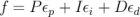where P, I and D are user inputs;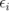and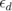are the integral and differential error associated with the residual.

The error is calculated as: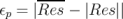where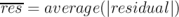.

To calculate the blended field between 0-1 we use the following linear dependency: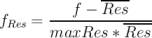where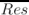is the average of the residuals in the domain and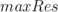is an user input.

Here,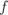tends to one when the difference (f -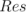) is maxResidual times meanRes. In other words, when a cell error is much larger of magnitude larger than the average the factor adds weight toward blending factor one. Residuals vary in time/iteration so the PID will dynamically change the weighting factors and will reduce or increase the weighting depending on the evolution of the residual.

In order to use this option, the function object needs the residual field, which is provided by the new residuals function object. For most cases the residual for pressure should be used.

For the other options please refer to the source code link below.

The overall cell-based blending factor field (f) is obtained from the individual error measures: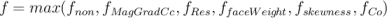An indicator (volume) field, named blendedIndicator is generated if the log flag is activated:

• 1: scheme1 as active,
• 0: scheme2 as active
• 0-1 is blended.

The cell-based blending factor field (f) finally gets interpolated to create the weight field for the localBlended scheme. Here it can be beneficial to use the localMax interpolation method to preserve the more stable choice, i.e. the take the value from the cell with the highest value.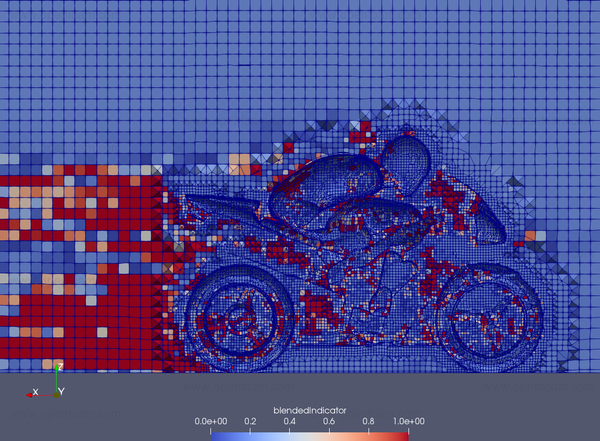Source code
$FOAM_SRC/functionObjects/field/stabilityBlendingFactor Examples$FOAM_TUTORIALS/incompressible/pisoFoam/LES/motorBike/motorBike